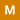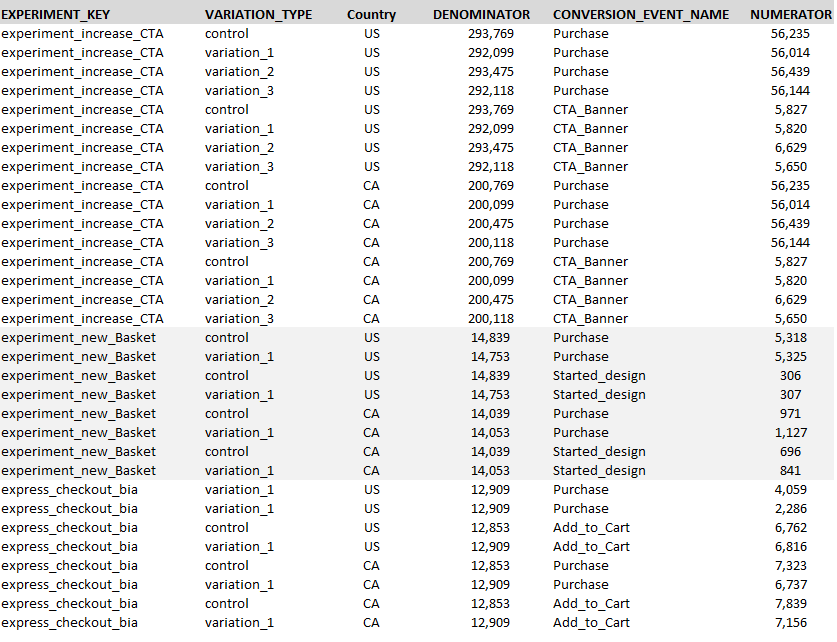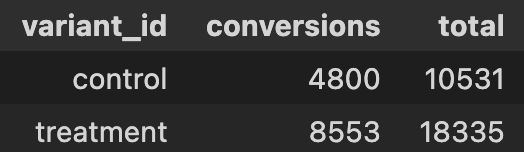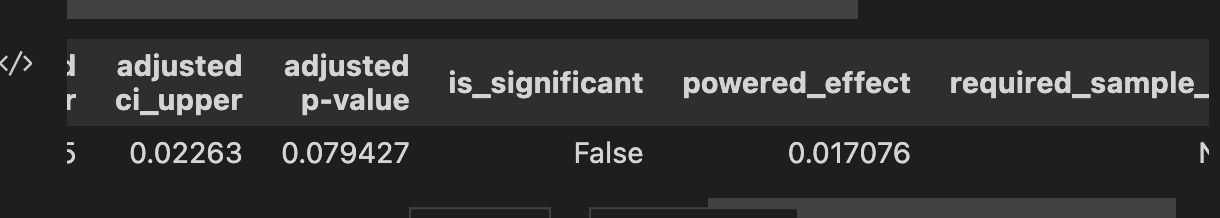spotify, updated 🕥 2023-03-30 11:03:37

# Spotify ConfidencePython library for AB test analysis.

## Why use Spotify Confidence?

Spotify Confidence provides convinience wrappers around statsmodel's various functions for computing p-values and confidence intervalls. With Spotify Confidence it's easy to compute several p-values and confidence bounds in one go, e.g. one for each country or for each date. Each function comes in two versions: - one that return a pandas dataframe, - one that returns a Chartify chart.

Spotify Confidence has support calculating p-values and confidence intervals using Z-statistics, Student's T-statistics (or more exactly Welch's T-test), as well as Chi-squared statistics. It also supports a variance reduction technique based on using pre-exposure data to fit a linear model.

There is also a Bayesian alternative in the BetaBinomial class.

## Examples

``` import spotify_confidence as confidence import pandas as pd

data = pd.DataFrame( {'variation_name': ['treatment1', 'control', 'treatment2', 'treatment3'], 'success': [50, 40, 10, 20], 'total': [100, 100, 50, 60] } )

test = confidence.ZTest( data, numerator_column='success', numerator_sum_squares_column=None, denominator_column='total', categorical_group_columns='variation_name', correction_method='bonferroni')

test.summary() test.difference(level_1='control', level_2='treatment1') test.multiple_difference(level='control', level_as_reference=True)

test.summary_plot().show() test.difference_plot(level_1='control', level_2='treatment1').show() test.multiple_difference_plot(level='control', level_as_reference=True).show() ```

See jupyter notebooks in `examples` folder for more complete examples.

## Installation

Spotify Confidence can be installed via pip:

`pip install spotify-confidence`

Find the latest release version here

### Code of Conduct

This project adheres to the Open Code of Conduct By participating, you are expected to honor this code.

## Issues

### [Snyk] Fix for 2 vulnerabilities

opened on 2023-03-28 19:06:50 by perploug

This PR was automatically created by Snyk using the credentials of a real user.

### Snyk has created this PR to fix one or more vulnerable packages in the `pip` dependencies of this project.

#### Changes included in this PR

• Changes to the following files to upgrade the vulnerable dependencies to a fixed version:
• requirements_dev.txt

#### Vulnerabilities that will be fixed

##### By pinning:

Severity | Priority Score () | Issue | Upgrade | Breaking Change | Exploit Maturity :-------------------------:|-------------------------|:-------------------------|:-------------------------|:-------------------------|:-------------------------| 531/1000
Why? Proof of Concept exploit, Has a fix available, CVSS 4.2 | Remote Code Execution (RCE)
SNYK-PYTHON-IPYTHON-3318382 | `ipython:`
`7.34.0 -> 8.10.0`
| No | Proof of Concept|
509/1000
Why?* Has a fix available, CVSS 5.9 | Regular Expression Denial of Service (ReDoS)
SNYK-PYTHON-SETUPTOOLS-3180412 | `setuptools:`
`39.0.1 -> 65.5.1`
| No | No Known Exploit

(*) Note that the real score may have changed since the PR was raised.

Some vulnerabilities couldn't be fully fixed and so Snyk will still find them when the project is tested again. This may be because the vulnerability existed within more than one direct dependency, but not all of the affected dependencies could be upgraded.

Check the changes in this PR to ensure they won't cause issues with your project.

Note: You are seeing this because you or someone else with access to this repository has authorized Snyk to open fix PRs.

For more information:🧐 View latest project report

Learn how to fix vulnerabilities with free interactive lessons:

### Dropped support for python 3.6

opened on 2023-03-24 12:46:26 by iampelle None

### Is possible to run the Ztest class and multiple_difference() method for multiple experiments and events at the same time?

opened on 2023-02-23 18:15:13 by fdesouza-git

We are considering using Spotify confidence to report on all the experiments running on our experimentation platform. So, I did some tests by running a sample of our data (see image below) against Ztest class to see if it could be used to meet our needs of running it simultaneously for various experiments and conversion events. And my findings were as follows:• For a Single Experiment (Variation_Type, Conversion_Event_Name)

For a single experiment with multiple metrics, the following methods, summary(), difference(), and multiple_difference(), worked correctly.

``` ztest_filtered = confidence.ZTest(pandasDF_filtered, numerator_column='NUMERATOR', numerator_sum_squares_column=None, denominator_column='DENOMINATOR', categorical_group_columns= ['VARIATION_TYPE','CONVERSION_EVENT_NAME'], interval_size=0.95, correction_method='bonferroni', #metric_column = 'CONVERSION_EVENT_NAME', )

ztest_filtered.summary() ztest_filtered.difference(level_1="control", level_2="variation_1", groupby="CONVERSION_EVENT_NAME", absolute=False) ztest_filtered.multiple_difference(level='control', groupby='CONVERSION_EVENT_NAME', level_as_reference=True)

```

• For a Multiple Experiments and conversion_Eevnts by making use of concatenation (Variation_Type, "Experiment_Key~Conversion_Event_Name")

Similar results to the previous one, but satisfying to see that it works perfectly for all experiments and events if we do a concatenation between the fields "Experiment_Key~Conversion_Event_Name".

``` ztest_concat = confidence.ZTest(pandasDF_updated, numerator_column='NUMERATOR', numerator_sum_squares_column='NUMERATOR', denominator_column='DENOMINATOR', categorical_group_columns=['VARIATION_TYPE','EXP_n_EVENT'], #ordinal_group_column = , interval_size=0.95, correction_method='bonferroni', #metric_column = 'CONVERSION_EVENT_NAME', #treatment_column , # power - 0.8 (default) )

ztest_concat.summary() ztest_concat.difference(level_1="control", level_2="variation_1", groupby="EXP_n_EVENT", absolute=False) ztest_concat.multiple_difference(level='control', groupby='EXP_n_EVENT', level_as_reference=True) ```

• **For all experiments using the above table as it is. (Experiment_Key, Variation_Type, Country, Conversion_Event_Name)

The summary class works even if I change the conversion_event from the categorical group to metric_column. While the methods difference () and multiple_difference() return errors regardless of the combinations, I can try in both the class and the method.

``` Trial 1: metric_column equals conversion_event_name

ztest = confidence.ZTest(pandasDF_updated, numerator_column='NUMERATOR', numerator_sum_squares_column='NUMERATOR', denominator_column='DENOMINATOR', categorical_group_columns=['VARIATION_TYPE','EXPERIMENT_KEY'], #ordinal_group_column = , interval_size=0.95, correction_method='bonferroni', metric_column = 'CONVERSION_EVENT_NAME', #treatment_column , # power - 0.8 (default) )

Trial 2 : metric_column hidden and conversion_event_name moved to categorical_group_columns

ztest = confidence.ZTest(pandasDF_updated, numerator_column='NUMERATOR', numerator_sum_squares_column='NUMERATOR', denominator_column='DENOMINATOR', categorical_group_columns=['VARIATION_TYPE','EXPERIMENT_KEY','CONVERSION_EVENT_NAME'], #ordinal_group_column = , interval_size=0.95, correction_method='bonferroni', #metric_column = 'CONVERSION_EVENT_NAME', #treatment_column , # power - 0.8 (default) )

```

`ztest.multiple_difference(level='control', groupby=['EXPERIMENT_KEY','CONVERSION_EVENT_NAME'], level_as_reference=True)` ValueError: cannot handle a non-unique multi-index! (for both trials)

I've been searching inside the repository notebooks, but I couldn't find the place that explains or execute this error message.

So after this test, I wondered:

1. Is there any configuration between the class and the method that meets our needs?
2. what is the use case for the variable "metric_column "?
3. at which level the "correction_method='bonferroni' " is applied?

Thanks, and looking forward to leveraging this package.

### `powered_effect` is not calculated in the `StudentsTTest`

opened on 2023-01-10 20:48:46 by jpzhangvincent

I'm a bit confused why the `powered_effect` is not calculated in the `StudentsTTest` but it's provided in `ZTest`.The above is the data frame which I passed into both ```stat_res_df = confidence.ZTest( stats_df, numerator_column='conversions', numerator_sum_squares_column=None, denominator_column='total', categorical_group_columns='variant_id', correction_method='bonferroni')```
and ```stat_res_df = confidence.StudentsTTest( stats_df, numerator_column='conversions', numerator_sum_squares_column=None, denominator_column='total', categorical_group_columns='variant_id', correction_method='bonferroni')```

but when I called `stat_res_df.difference(level_1='control', level_2='treatment')` I found the result from z-test provides the `powered_effect` column as belowbut it's missing from the t-test result. Another question, why is the `required_sample_size` missing? Is there a way to also provide the sample size estimation in the result? Thanks!

### [Question] What is the Powered effect in the results?

opened on 2022-09-24 22:52:10 by LibiSC

If you could tell me. I can't fully understand it from the code

### Tanking tests

opened on 2022-02-21 21:12:07 by ankargren

PR for adding support for tanking tests. Some things remain.

## Releases

### Release 2.7.7 2023-01-22 09:25:38

• Fixed bug that led to unexpected behaviour when using non_inferiority_margins=False. Not passing False produces the same result as passing None
• Fixed bug in chartify grapher that caused a crash when attempting to plot a mix of metrics where only some had non-inferiority margins

### Release 2.7.6 2022-11-23 07:37:53

• Fixed bug in compute_sequential_adjusted_alpha where we since 2.7.4 were taking the max sample size rowwise

### Release 2.7.5 2022-11-15 22:52:17

• Major refactoring, splitting the code into more files for improved readability
• Fixed bugs related to group sequential testing that resulted in to narrow confidence bands for tests with multiple treatment groups
• Bump Chartify version
• Minor changes to get rid of warnings

### Release 2.7.4 2022-08-31 15:14:29

Fixed bug in sample size calculator check for binary metrics when there are nans

### Release 2.7.3 2022-08-29 15:38:20

• Fixed bug in SampleSizeCalculator.optimal_weights_and_sample_size.

• Added check to make point estimates and variances match for binary metrics when using the sample size calculator.

### Release 2.7.2 2022-08-18 08:56:11

• Adding a constant to the variance reduction point estimate so that the adjusted value is close in magnitude to the original value.
• Changing `level_as_reference` to default to `None` in `multiple_difference_plot` to be consistent with `multiple_difference`
• Updated docstrings relating to the default value of `level_as_reference`